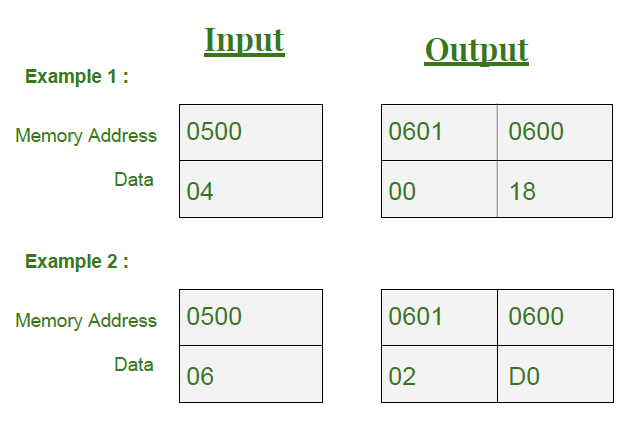# 8086 program to find the factorial of a number

• Difficulty Level : Medium
• Last Updated : 22 May, 2018

Prerequisite – 8085 program to find the factorial of a number
Problem – Write an assembly language program for calculating the factorial of a number using 8086 microprocessor

Examples –

```Input : 04H
Output : 18H
as In Decimal : 4*3*2*1 = 24
In Hexadecimal : 24 = 18H

Input : 06H
Output : 02D0H
as In Decimal : 6*5*4*3*2*1 = 720
In Hexadecimal : 720 = 02D0H```

Assumptions –
Input memory location: 0500
Output memory location: 0600 and 0601

Important –
If the Given Number is a 16-bit number, the AX register is automatically used as the second parameter and the product is stored in the DX:AX register pair. This means that the DX register holds the high part and the AX register holds the low part of a 32-bit number.In 8086 microprocessor, user have direct instruction (MUL) to multiply two numbers, so we don’t have to add Multiplicand by Multiplier times like in 8085

Advantage of 8086 over 8085 (In case of Multiply):

• Don’t have to write a bulky code as 8086 has a small code
• Easy to remember

Algorithm –

1. Input the Number whose factorial is to be find and Store that Number in CX Register (Condition for LOOP Instruction)
2. Insert 0001 in AX(Condition for MUL Instruction) and 0000 in DX
3. Multiply CX with AX until CX become Zero(0) using LOOP Instruction
4. Copy the content of AX to memory location 0600
5. Copy the content of DX to memory location 0601
6. Stop Execution

Program –

0400 MOV CX,  CX <- 
0404 MOV AX, 0001 AX <- 0001
0407 MOV DX, 0000 DX <- 0000
040A MUL CX DX:AX <- AX * CX
040C LOOP 040A Go To [040A] till CX->00
0410 MOV , AX <-AX
0414 MOV , DX <-DX
0418 HLT Stop Execution

Explanation –

1. MOV CX,  loads 0500 Memory location content to CX Register
2. MOV AX, 0001 loads AX register with 0001
3. MOV DX, 0000 loads DX register with 0000
4. MUL CX multiply AX with CX and store result in DX:AX pair
5. LOOP 040A runs loop till CX not equal to Zero
6. MOV , AX store AX register content to memory location 0600
7. MOV , DX store DX register content to memory location 0601
8. HLT stops the execution of program
My Personal Notes arrow_drop_up
Recommended Articles
Page :